## Permutations and Combinations Questions and Answers Part-1

1. Number of solutions of $2x^{\left(x!+x\right)}=\left(x!+x\right)^{x},x\epsilon N$
is
a) 0
b) 1
c) 2
d) infinite

Explanation: For x $\epsilon$ N, we can write the given equation as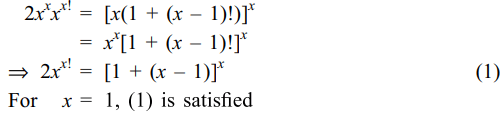2. Let n = 2019. The least positive integer k for which
$k\left(n^{2}\right)\left(n^{2}-1^{2}\right)\left(n^{2}-2^{2}\right)\left(n^{2}-3^{2}\right)....\left(n^{2}-\left(n-1\right)^{2}\right)=r!$
for some positive integer r is
a) 2019
b) 2018
c) 1
d) 2

Explanation: We can rewrite the given expression as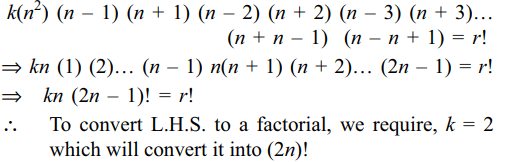3. If $0< r < s\leq n$    and $^{n}P_{r}=^{n}P_{s}$    , then value of r + s is
a) 2n – 2
b) 2n – 1
c) 2
d) 1

Explanation: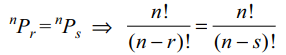4. if $\frac{1}{4}.\frac{2}{6}.\frac{3}{8}.\frac{4}{10}....\frac{30}{62}.\frac{31}{64}=8^{x}$
then value of x is
a) -7
b) -9
c) -10
d) -12

Explanation:5. The sum$\sum_{i=0}^{m}\left(\begin{array}{c}10\\ i\end{array}\right)\left(\begin{array}{c}20\\ m-i\end{array}\right)$     (where $\left(\begin{array}{c}p\\ q\end{array}\right)=0$     if (p < q) is maximum where m is
a) 5
b) 10
c) 15
d) 20

Explanation: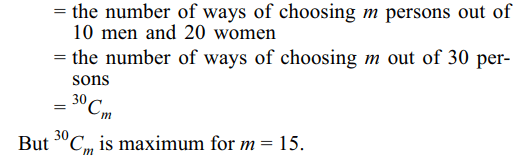6. Let $T_{n}$ denote the number of triangles which can be formed by using the vertices of a regular polygon of n sides. If $T_{n+1}-T_{n}=21$    , then n equals
a) 5
b) 7
c) 6
d) 4

Explanation: The number of triangles that can be formed by7. An eight digits number divisible by 9 is to be formed by using 8 digits out of the digits 0, 1, 2, 3, 4, 5, 6, 7, 8, 9 without replacement. The number of ways in which this can be done is
a) 9!
b) 2(7!)
c) 4(7!)
d) (36) (7!)

Explanation: We have 0 + 1 + 2 + 3 … + 8 + 9 = 45
To obtain an eight digits number exactly divisible by 9, we must not use either (0, 9) or (1, 8) or (2, 7) or (3, 6) or (4, 5). [Sum of the remaining eight digits is 36 which is exactly divisible by 9].
When, we do not use (0, 9), then the number of required 8 digit number is 8!.
When one of (1, 8) or (2, 7) or (3, 6) or (4, 5) is not used, the remaining digits can be arranged in 8! – 7! ways as 0 cannot be at extreme left.
Hence, there are 8! + 4(8! – 7!) = (36) (7!) numbers in the desired category

8. The number of rational numbers lying in the interval (2018, 2019) all whose digits after the decimal point are non-zero and are in decreasing order is
a) $\sum_{i=1}^{9}$  $^{9}P_{i}$
b) $\sum_{i=1}^{10}$  $^{9}P_{i}$
c) $2^{9}-1$
d) $2^{10}-1$

Explanation: A rational number of the desired category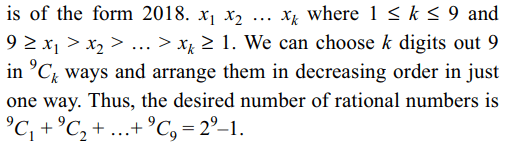9. The number of functions f from the set A = {0, 1, 2} in to the set B = {0, 1, 2, 3, 4, 5, 6, 7} such that $f\left(i\right)\leq f\left(j\right)$   for i < j and $i,j \epsilon A$   is
a) $^{8}C_{3}$
b) $^{8}C_{3}+2\left(^{8}C_{2}\right)$
c) $^{10}C_{3}$
d) $^{9}C_{3}$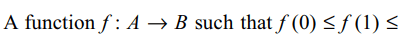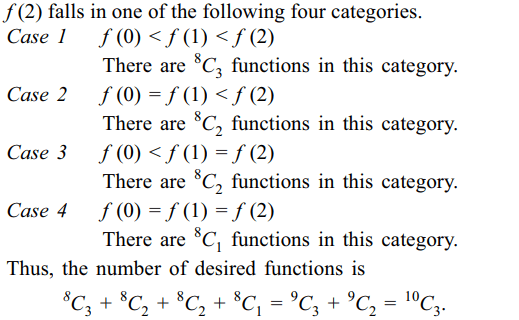10. The number of positive integral solutions of the equation $x_{1}x_{2}x_{3}x_{4}x_{5}=1050$      is